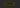# How to Implement a FizzBuzz solution in JavaScriptFizzBuzz question is most commonly asked in interviews to test the problem-solving skills.

Write a program that prints the numbers from 1 to 100. But for multiples of three print “Fizz” instead of the number and for the multiples of five print “Buzz”. For numbers which are multiples of both three and five print “FizzBuzz”.

### Solution

``````let i = 1;

while (i <= 100) {
console.log(i % 15 ? (i % 5 ? (i % 3 ? i : 'fizz') : 'buzz') : 'fizzbuzz');
i = i + 1;
}``````

Let’s break down the problem into 4 steps.

1. Print the numbers from 1 to 100.
2. Multiples of 3 we need to print the word “fizz” instead of a number.
3. Multiples of 5 we need to print the word “buzz” instead of number.
4. Multiples of 3 and 5 we need to print “fizzbuzz” instead of a number.

### What are the Multiples of 3?

Any number that can be divided by 3 and produces remainder 0.

`[3,6,9,12,15,18...]` are the multiples of 3.

In JavaScript, we can use the modulo(%) operator to get the remainder of the number.

``````9 % 3 = 0;

// where number1 is dividen` and number2 is a divisor

// 0 is the remainder.``````

Exactly we can also find the multiples of 5 using modulo(%) operator.

``````10 % 5 = 0;

// 10 is a multiple of 5``````

### Multiplies of 3 and 5

There are certain numbers which can be multiples of both 3 and 5.

``````15 % 3 = 0;

15 % 5 = 0;``````

Instead of finding multiples of both 3 and 5 we can only find multiples of 15 and print “fizzbuzz”.

Let’s write the solution by using the while loop and Ternary Operator.

Ternary Operator

``````condition ? true : false;

1 === 1 ? true : false; // true

9 % 3 ? 1 : 'fizz'; // "fizz".

10 % 5 ? 5 : 'buzz'; // "buzz"``````

The whole fizzbuzz solution uses the ternary operator to print the correct output.

## Fizz Buzz

``````let i = 1;

while (i <= 100) {
console.log(i % 15 ? (i % 5 ? (i % 3 ? i : 'fizz') : 'buzz') : 'fizzbuzz');
i = i + 1;
}``````

## How above code works?

1. We created a while loop which helps us to produce 1 to 100 numbers.
2. Inside the while loop. The condition is `i % 15 ? i % 5 ? i % 3 ? i : "fizz" : "bizz": "fizzbuzz"`
``````if i % 15 returns 0(false) it means we found multiples of 15(3 and 5) so
we printed "fizzbuzz"

if i % 15 returns true it means no multiples so we check for

`i % 5 ? i % 3 ? i : "fizz" : "bizz"```````

There are many ways to solve this problem but in this tutorial, we have seen the shortest possible solution.

happy coding..

## Top Udemy Courses##### JavaScript - The Complete Guide 2020 (Beginner + Advanced)
45,614 students enrolled
52 hours of video content
View Course##### React - The Complete Guide (incl Hooks, React Router, Redux)
284,472 students enrolled
40 hours of video content
View Course##### Vue JS 2 - The Complete Guide (incl. Vue Router & Vuex)
130,921 students enrolled
21 hours of video content
View Course